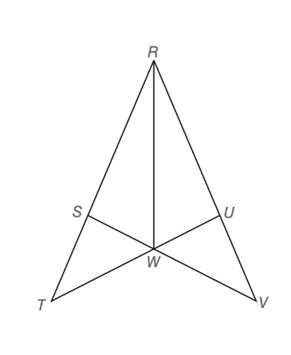Chapter 3.2, Problem 33E### Elementary Geometry for College St...

6th Edition
Daniel C. Alexander + 1 other
ISBN: 9781285195698

#### Solutions

Chapter
Section### Elementary Geometry for College St...

6th Edition
Daniel C. Alexander + 1 other
ISBN: 9781285195698
Textbook Problem
50 views

# Given: R W → bisects ∠ S R U Prove: R S ¯ ≅ R U ¯ Δ T R U ≅ Δ V R S (HINT: First show that Δ R S W ≅ Δ R U W .)To determine

To prove:

The given statement.

Explanation

Given:

The given statements are,

RW bisects SRU and RS¯RU¯

Figure (1)

Properties used:

(1) If two sides and the included angle between the sides of two triangles are equal, then the triangles are congruent.

(2) If two angles and one side of two triangles are equal, then the triangles are congruent.

(3) If two triangles are congruent to each other then all sides of the triangle are congruent.

Approach:

The first given statement is,

RW bisects SRU

SRW and URW are the bisected angles.

So,

SRWURW

The given statement is,

RS¯RU¯

The side RW is common in both triangles ΔMQP and ΔPNM.

So,

RW¯RW¯

Use SAS criteria of triangle congruency.

RS¯RU¯

SRWURW

RW¯RW¯

So,

ΔRSWΔRUW

Use transitive property of congruence.

ΔRSWΔRUW and RSW, and RUW are the angles of congruent triangles

### Still sussing out bartleby?

Check out a sample textbook solution.

See a sample solution

#### The Solution to Your Study Problems

Bartleby provides explanations to thousands of textbook problems written by our experts, many with advanced degrees!

Get Started

#### In Exercises 73-80, find the indicated limits, if they exist. 75. limx3x3+x2+1x3+1

Applied Calculus for the Managerial, Life, and Social Sciences: A Brief Approach

#### Change 40 mi/h to ft/s.

Elementary Technical Mathematics

#### Define and compute the three measures of central tendency.

Research Methods for the Behavioral Sciences (MindTap Course List)

#### Explain why partial counterbalancing is sometimes necessary.

Research Methods for the Behavioral Sciences (MindTap Course List)